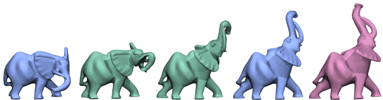Geometric Modeling in Shape Space
Martin Kilian, Niloy J. Mitra, Helmut Pottmann
ACM SIGGRAPH 2007

Abstract:

We present a novel framework to treat shapes in the setting of Riemannian geometry. Shapes - triangular meshes or more generally straight line graphs in Euclidean space - are treated as points in a shape space. We introduce useful Riemannian metrics in this space to aid the user in design and modeling tasks, especially to explore the space of (approximately) isometric deformations of a given shape. Much of the work relies on an efficient algorithm to compute geodesics in shape spaces; to this end, we present a multiresolution framework to solve the interpolation problem - which amounts to solving a boundary value problem - as well as the extrapolation problem - an initial value problem - in shape space. Based on these two operations, several classical concepts like parallel transport and the exponential map can be used in shape space to solve various geometric modeling and geometry processing tasks. Applications include shape morphing, shape deformation, deformation transfer, and intuitive shape exploration.

Result video:

Bibtex:

```@article{kmp_shape_space_sig_07,
AUTHOR = "Martin Kilian and Niloy J. Mitra and Helmut Pottmann",
TITLE = "Geometric Modeling in Shape Space",
JOURNAL = "ACM Transactions on Graphics (SIGGRAPH)",
VOLUME = "26",
NUMBER = "3",
PAGES = "\#64, 1-8",
YEAR = "2007",
}```

Data Repository:

Input data sets used in the paper are available here.